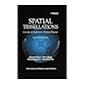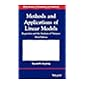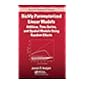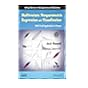Normal view MARC view ISBD view

# Methods and applications of linear models: regression and the analysis of variance

Publisher: New Jersey John Wiley & Sons 2013Edition: 3rd ed.Description: xxiii, 676 p.ISBN: 9781118329504.DDC classification: 519.536 Summary: The book presents a unique discussion that combines coverage of mathematical theory of linear models with analysis of variance models, providing readers with a comprehensive understanding of both the theoretical and technical aspects of linear models. With a new focus on fixed effects models, Methods and Applications of Linear Models: Regression and the Analysis of Variance, Third Edition also features: Newly added topics including least squares, the cell means model, and graphical inspection of data in the AVE method Frequent conceptual and numerical examples for clarifying the statistical analyses and demonstrating potential pitfalls Graphics and computations developed using JMP software to accompany the concepts and techniques presented Numerous exercises presented to test readers and deepen their understanding of the material An ideal book for courses on linear models and linear regression at the undergraduate and graduate levels, the Third Edition of Methods and Applications of Linear Models: Regression and the Analysis of Variance is also a valuable reference for applied statisticians and researchers who utilize linear model methodology.
Tags from this library: No tags from this library for this title.
Item type Current location Item location Collection Call number Status Date due Barcode
Books Vikram Sarabhai Library
Slot 1427 (0 Floor, East Wing) Non-fiction 519.536 H6M3 (Browse shelf) Available 180277
##### Browsing Vikram Sarabhai Library Shelves , Collection code: Non-fiction Close shelf browser519.5​36 O5S7/2000 Spatial tessellations: concepts and applications of voronoi diagrams 519.536 H6M3 Methods and applications of linear models: regression and the analysis of variance 519.536 H6R4 Richly parameterized linear models: additive, time series, and spatial models using random effects 519.536 K3M8 Multiple regression and beyond: an introduction to multiple regression and structural equation modeling 519.536 K5M8 Multivariate nonparametric regression and visualization: with R and applications to finance

The book presents a unique discussion that combines coverage of mathematical theory of linear models with analysis of variance models, providing readers with a comprehensive understanding of both the theoretical and technical aspects of linear models. With a new focus on fixed effects models, Methods and Applications of Linear Models: Regression and the Analysis of Variance, Third Edition also features:

Newly added topics including least squares, the cell means model, and graphical inspection of data in the AVE method
Frequent conceptual and numerical examples for clarifying the statistical analyses and demonstrating potential pitfalls
Graphics and computations developed using JMP software to accompany the concepts and techniques presented
Numerous exercises presented to test readers and deepen their understanding of the material

An ideal book for courses on linear models and linear regression at the undergraduate and graduate levels, the Third Edition of Methods and Applications of Linear Models: Regression and the Analysis of Variance is also a valuable reference for applied statisticians and researchers who utilize linear model methodology.

There are no comments for this item.